Next: Waves Up: Homework Problems for PHYS262, Previous: Oscillations

# Fluids

2-1
An office window is 3.4 m by 2.1 m. As a result of the passage of a storm, the outside air pressure drops to 0.96 atm, but inside the pressure is held at 1.0 atm. What net force pushes out on the window?

2-2
what is the total mass of the earth's atmosphere? (The radius of the earth is
, and atmospheric pressure at Earth's surface is
.)

2-3
Figure 1 displays the phase diagram of carbon, showing the range of temperature and pressure in which carbon will crystallise either as diamond or graphite. What is the minimum depth at which diamonds can form if the local temperature is
and the subsurface rocks have density
? Assume that, as in fluid, the pressure is due to the weight of material lying above.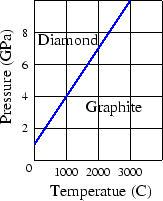2-4
(a) Find the total weight of water on top of a nuclear submarine at a depth of 200 m, assuming that its (cross-sectional) hull area is
. (b)What water pressure would a diver experience at this depth? Express your answer in atmospheres. Do you think that occupants of a damaged submarine at this depth could escape without special equipment? The density of sea water is
.

2-5
In analysing certain geological features of the earth, it is often appropriate to assume that the pressure at some horizontal level of compensation, deep in the earth, is the same over a large region and is equal to that exerted by the weight of the overlying material. That is, the pressure on the level of compensation is given by the hydrostatic (fluid) pressure formula. This requires, for example, that mountains have low-density roots; see Fig. 2. Consider a mountain
high. The continental rocks have a density of
; beneath the continent is the mantle, with a density of
. Calculate the depth
of the root. (Hint: Set the pressure at points
and
equal; the depth
of the level of compensation will cancel out.)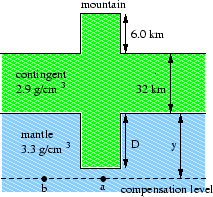2-6
A U-tube of uniform cross-sectional area and open to the atmosphere is partially filled with mercury. Water is then poured into both arms. If the equilibrium configuration of the tube is as shown in Fig. 3, with
, determine the value
.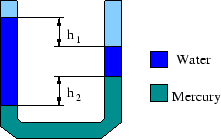2-7
A helium balloon is used to lift a 40-kg payload to an altitude of 27 km, where the air density is
. The balloon has a mass of 15 kg and the density of the gas in the balloon is
. What is the volume of the balloon? Neglect the volume of the payload.

2-8
A piece of aluminium with mass 1.00 kg and the density
is suspended from a string and then completely immersed in a container of water. Calculate the tension in the string (a) before and (b) after the metal is immersed.

2-9
Models of torpedoes are sometimes tested in a horizontal pipe of flowing water, much as a wind tunnel is used to test model airplanes. Consider a circular pipe of internal diameter 25 cm and a torpedo model, aligned along the axis of the pipe, with a diameter of 5.0 cm. The torpedo is to be tested with water flowing past it at 2.5 m/s. (a) With what speed must the water flow in the unconstricted part of the pipe? (b) What will the pressure difference be between the constricted and unconstricted parts of the pipe?

2-10
Air flows over the top of an airplane wing, area
, with speed
and past the underside of the wing with speed. Show that Bernoulli's equation predicts that the upward lift force
on the wing will be
where
is the density of the air.

2-11
A syphon is a device for removing liquid from a container that cannot be tipped. It operates as shown in Fig. 4. The tube must initially be filled, but once this has been done the liquid will flow until its level drops below the tube opening at. The liquid has densityand negligible viscosity. (a) With what speed does the liquid emerge from the tube at
? (b) What is the pressure in the liquid at the topmost point
? (c) What is the greatest possible heightthat a syphon can lift water?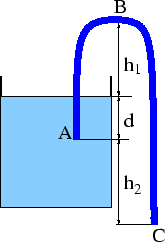Next: Waves Up: Homework Problems for PHYS262, Previous: Oscillations
HJK 2001-07-22